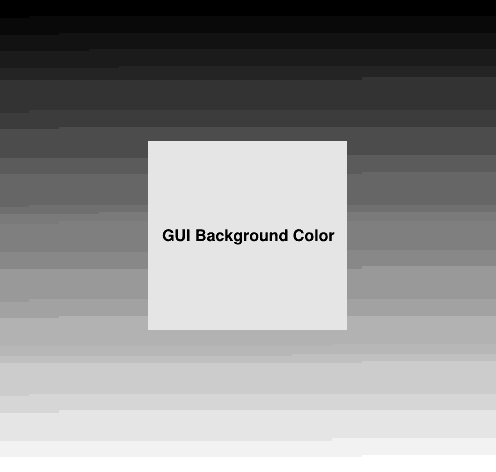# Greyscale¶

Create grey scale of 200 x 200 boxes.

Author: Olivier Couet
This notebook tutorial was automatically generated with ROOTBOOK-izer from the macro found in the ROOT repository on Thursday, May 19, 2022 at 07:55 AM.

In :
TCanvas *c = new TCanvas("grey", "Grey Scale", 500, 500);
c->SetBorderMode(0);

Int_t   n = 200;   // tunable parameter
Float_t n1 = 1./n;
for (int i = 0; i < n; i++) {
for (int j = 0; j < n; j++) {
TBox *b = new TBox(n1*j, n1*(n-1-i), n1*(j+1), n1*(n-i));
Float_t grey = Float_t(i*n+j)/(n*n);
b->SetFillColor(TColor::GetColor(grey, grey, grey));
b->Draw();
}
}
const char *guibackground = gEnv->GetValue("Gui.BackgroundColor", "");
p->SetFillColor(TColor::GetColor(guibackground));
p->Draw();
p->cd();
TText *t = new TText(0.5, 0.5, "GUI Background Color");
t->SetTextAlign(22);
t->SetTextSize(.09);
t->Draw();

c->SetEditable(kFALSE);


Draw all canvases

In :
gROOT->GetListOfCanvases()->Draw()